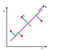# Principal Component Analysis- The MATH

Hi, Isn’t PCA a hyped method for Dimensionality Reduction. All for good reasons. Let’s look out what maths has to say about this Non Parametric Method.

• Principal component analysis (PCA) Orthogonally transforms the original n numeric dimensions of a dataset into a new set of m dimensions called principal components. As a result of the transformation, the first principal component has the largest possible variance; each succeeding principal component has the highest possible variance under the constraint that it is orthogonal to (i.e., uncorrelated with) the preceding principal components. Keeping only the first m < n principal components reduces the data dimensionality while retaining most of the data information, i.e., variation in the data.

Below is formulation of PCA

Project entire D dimensional data onto K<D space, where K are orthogonal components of Covariance matrix(derived below).Fig 1
1. Minimize projection error

2. Maximize the Variance of projected Data

Note Theoretically both are equivalent.

Note, only direction of u_1 matters to us, so we can restrict its magnitude/norm to be 1. By using fig1, On projecting vector x_{i} onto u_{1}, and calculating variance of projected data.

This essentially means that u_{1} is the eigen vector of Covariance Matrix(Sigma).Lambda_1 implying variance is maximized by choosing the eigenvector associated with the largest eigenvalue. This u_1 corresponds to the first Principal component.

Now we find second component by again maximizing variance subject to magnitude of it being 1 and it being perpendicular to already found out PC1(u_1).

This again leads to to eigen vector of Covariance matrix, corresponding to lambda_2, which should be second highest eigen value of Sigma matrix(Covariance matrix). And so on…we can find all principal components.

# MY SOCIAL SPACE

Problem Solver, Data Science, Actuarial Science, Knowledge Sharer, Hardcore Googler

## More from Shaily jain

Problem Solver, Data Science, Actuarial Science, Knowledge Sharer, Hardcore Googler